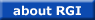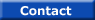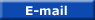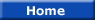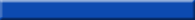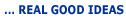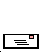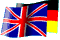### MEASURING PRINCIPLE

Electro-magnetic waves like X-rays or gamma-rays are absorbed in material by different ways. Therefore it depends  on the energy of the radiation and on the material itself in which way it is absorbed. High energy waves and low  atomic numbers are absorbed according to the density (or bulk density) of the material. Of course the absorption  also is depending on the layer thickness. That means the absorption is depending on the weight of the material  the radiation has passed through ( The weight increases if the way becomes longer or if the density becomes higher).  Density and thickness can be measured by means of radiations: If one is constant the other can be calculated.

Lower energy radiation and higher atomic numbers are absorbed in the same way but additional according to the  atomic weight. As higher the atomic weight and as lower the energy as higher the absorption.

For analysis purposes a weak energy is needed to determine the atomic composition. But also the weight is influencing  the absorption. So a higher energy radiation also must be applied to get a signal which is influenced by the weight  only but not by the atomic composition. Both signals can be used mathematically to determine the composition.

Tel.: 49 7081 5026  Fax: 49 7081 5526
Webhosting and design by RPF Netdesigncanal-meas